# mel705-22 (1).ppt

May. 29, 2023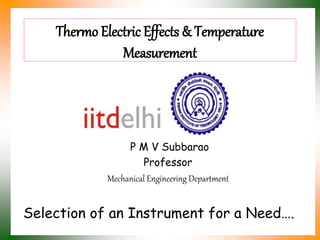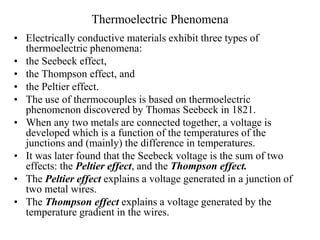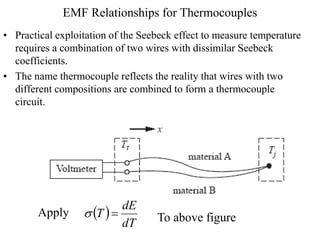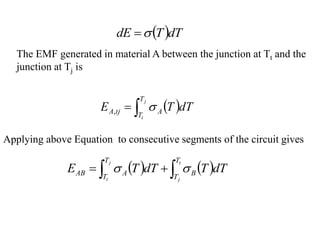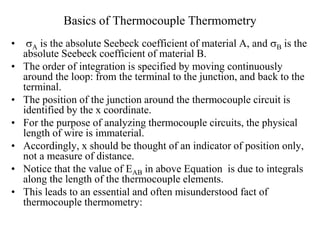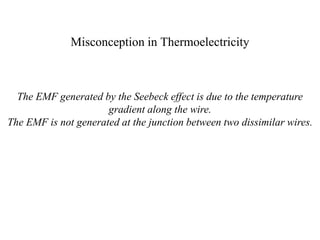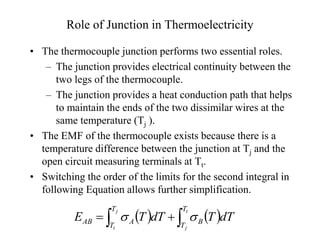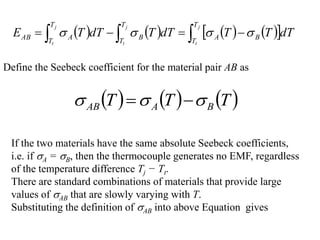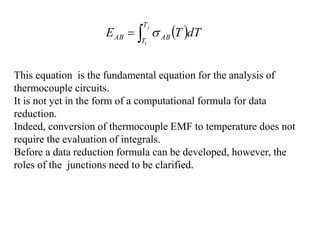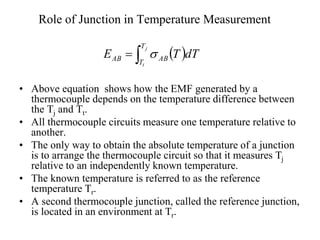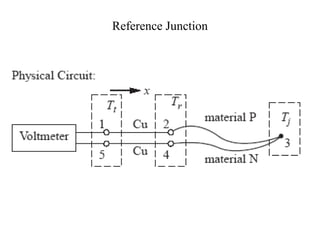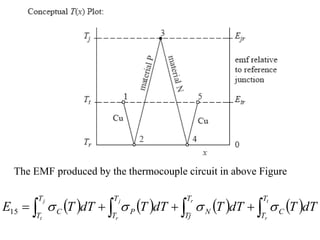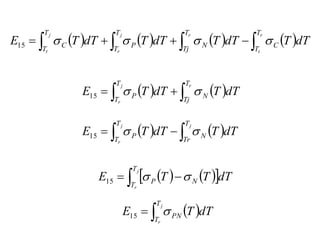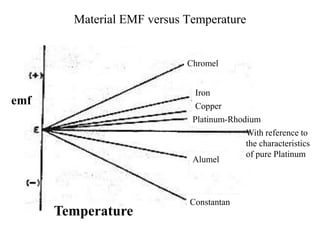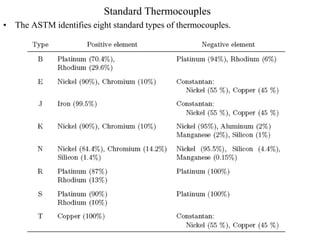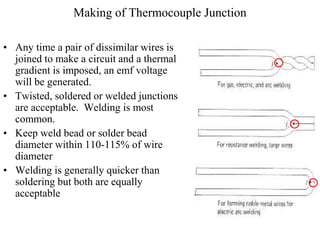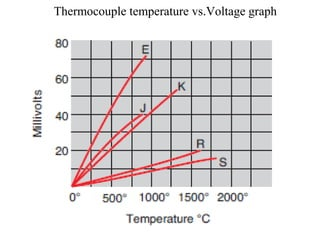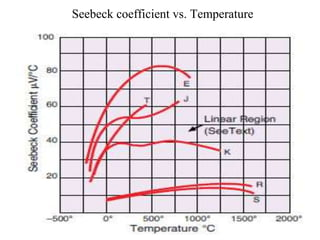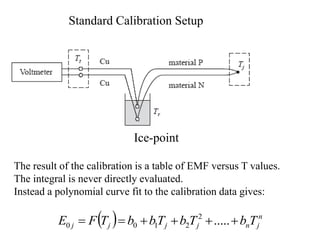1 of 19

### mel705-22 (1).ppt

• 1. Thermo Electric Effects & Temperature Measurement P M V Subbarao Professor Mechanical Engineering Department Selection of an Instrument for a Need….
• 2. Thermoelectric Phenomena • Electrically conductive materials exhibit three types of thermoelectric phenomena: • the Seebeck effect, • the Thompson effect, and • the Peltier effect. • The use of thermocouples is based on thermoelectric phenomenon discovered by Thomas Seebeck in 1821. • When any two metals are connected together, a voltage is developed which is a function of the temperatures of the junctions and (mainly) the difference in temperatures. • It was later found that the Seebeck voltage is the sum of two effects: the Peltier effect, and the Thompson effect. • The Peltier effect explains a voltage generated in a junction of two metal wires. • The Thompson effect explains a voltage generated by the temperature gradient in the wires.
• 3. EMF Relationships for Thermocouples • Practical exploitation of the Seebeck effect to measure temperature requires a combination of two wires with dissimilar Seebeck coefficients. • The name thermocouple reflects the reality that wires with two different compositions are combined to form a thermocouple circuit. Apply   dT dE T   To above figure
• 4.  dT T dE   The EMF generated in material A between the junction at Tt and the junction at Tj is     j t T T A tj A dT T E  , Applying above Equation to consecutive segments of the circuit gives         t j j t T T B T T A AB dT T dT T E  
• 5. Basics of Thermocouple Thermometry • A is the absolute Seebeck coefficient of material A, and B is the absolute Seebeck coefficient of material B. • The order of integration is specified by moving continuously around the loop: from the terminal to the junction, and back to the terminal. • The position of the junction around the thermocouple circuit is identified by the x coordinate. • For the purpose of analyzing thermocouple circuits, the physical length of wire is immaterial. • Accordingly, x should be thought of an indicator of position only, not a measure of distance. • Notice that the value of EAB in above Equation is due to integrals along the length of the thermocouple elements. • This leads to an essential and often misunderstood fact of thermocouple thermometry:
• 6. Misconception in Thermoelectricity The EMF generated by the Seebeck effect is due to the temperature gradient along the wire. The EMF is not generated at the junction between two dissimilar wires.
• 7. Role of Junction in Thermoelectricity • The thermocouple junction performs two essential roles. – The junction provides electrical continuity between the two legs of the thermocouple. – The junction provides a heat conduction path that helps to maintain the ends of the two dissimilar wires at the same temperature (Tj ). • The EMF of the thermocouple exists because there is a temperature difference between the junction at Tj and the open circuit measuring terminals at Tt. • Switching the order of the limits for the second integral in following Equation allows further simplification.         t j j t T T B T T A AB dT T dT T E  
• 8.                  j t j t j t T T B A T T B T T A AB dT T T dT T dT T E     Define the Seebeck coefficient for the material pair AB as       T T T B A AB      If the two materials have the same absolute Seebeck coefficients, i.e. if A = B, then the thermocouple generates no EMF, regardless of the temperature difference Tj − Tt. There are standard combinations of materials that provide large values of AB that are slowly varying with T. Substituting the definition of AB into above Equation gives
• 9.     j t T T AB AB dT T E  This equation is the fundamental equation for the analysis of thermocouple circuits. It is not yet in the form of a computational formula for data reduction. Indeed, conversion of thermocouple EMF to temperature does not require the evaluation of integrals. Before a data reduction formula can be developed, however, the roles of the junctions need to be clarified.
• 10. Role of Junction in Temperature Measurement • Above equation shows how the EMF generated by a thermocouple depends on the temperature difference between the Tj and Tt. • All thermocouple circuits measure one temperature relative to another. • The only way to obtain the absolute temperature of a junction is to arrange the thermocouple circuit so that it measures Tj relative to an independently known temperature. • The known temperature is referred to as the reference temperature Tr. • A second thermocouple junction, called the reference junction, is located in an environment at Tr.     j t T T AB AB dT T E 
• 12. The EMF produced by the thermocouple circuit in above Figure                 t r r j r j t T T C T Tj N T T P T T C dT T dT T dT T dT T E     15
• 13.                 r t r j r j t T T C T Tj N T T P T T C dT T dT T dT T dT T E     15         r j r T Tj N T T P dT T dT T E   15         j j r T Tr N T T P dT T dT T E   15          j r T T N P dT T T E   15     j r T T PN dT T E  15
• 14. Material EMF versus Temperature With reference to the characteristics of pure Platinum emf Temperature Chromel Iron Copper Platinum-Rhodium Alumel Constantan
• 15. Standard Thermocouples • The ASTM identifies eight standard types of thermocouples.
• 16. Making of Thermocouple Junction • Any time a pair of dissimilar wires is joined to make a circuit and a thermal gradient is imposed, an emf voltage will be generated. • Twisted, soldered or welded junctions are acceptable. Welding is most common. • Keep weld bead or solder bead diameter within 110-115% of wire diameter • Welding is generally quicker than soldering but both are equally acceptable
• 18. Seebeck coefficient vs. Temperature
• 19. Standard Calibration Setup Ice-point The result of the calibration is a table of EMF versus T values. The integral is never directly evaluated. Instead a polynomial curve fit to the calibration data gives:   n j n j j j j T b T b T b b T F E       ..... 2 2 1 0 0